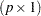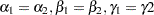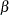## Example 68.7 Linear Inference with Arbitrary Estimates

Suppose that you have calculated a vector of parameter estimates of dimensionand its associated variance-covariance matrix by some statistical method. You are now interested in using these results to perform linear inference, or perhaps to score a data set and to calculate predicted values and their standard errors.

The following DATA steps create two SAS data sets. The first, called parms, contains six estimates that represent two uncorrelated groups. The data set cov contains the covariance matrix of the estimates. The lack of correlation between the two sets of three parameters is evident in the block-diagonal structure of the covariance matrix.

```data parms;
length name \$6;
input Name\$ Value;
datalines;
alpha1 -3.5671
beta1   0.4421
gamma1 -2.6230
alpha2 -3.0111
beta2   0.3977
gamma2 -2.4442
;
```
```data cov;
input Parm row col1-col6;
datalines;
1 1  0.007462 -0.005222  0.010234  0.000000  0.000000  0.000000
1 2 -0.005222  0.048197 -0.010590  0.000000  0.000000  0.000000
1 3  0.010234 -0.010590  0.215999  0.000000  0.000000  0.000000
1 4  0.000000  0.000000  0.000000  0.031261 -0.009096  0.015785
1 5  0.000000  0.000000  0.000000 -0.009096  0.039487 -0.019996
1 6  0.000000  0.000000  0.000000  0.015785 -0.019996  0.126172
;
```

Suppose that you are interested in testing whether the parameters are homogeneous across groups—that is, whether. You are interested in testing the hypothesis jointly and separately with multiplicity adjustment.

In order to use the facilities of the PLM procedure, you first need to create an item store that contains the necessary information as if the preceding parameter vector and covariance matrix were the result of a statistical modeling procedure. The following statements use the multivariate facilities of the GLIMMIX procedure to create such an item store, by fitting a saturated linear model with the GLIMMIX procedure where the data set that contains the parameter estimates serves as the input data set:

```proc glimmix data=parms order=data;
class Name;
model Value = Name / noint ddfm=none s;
random _residual_ / type=lin(1) ldata=cov v;
parms (1) / noiter;
store ArtificialModel;
title 'Linear Inference';
run;
```

The RANDOM statement is used to form the covariance structure for the estimates. The PARMS statement prevents iterative updates of the covariance parameters. The resulting marginal covariance matrix of the "data" is thus identical to the covariance matrix in the data set cov. The ORDER=DATA option in the PROC GLIMMIX statement is used to arrange the levels of the classification variable Name in the order in which they appear in the data set so that the order of the parameters matches that of the covariance matrix.

The results of this analysis are shown in Output 68.7.1. Note that the parameter estimates are identical to the values passed in the input data set and their standard errors equal the square root of the diagonal elements of the cov data set.

Output 68.7.1 "Fitted" Parameter Estimates and Covariance Matrix
 Linear Inference

The GLIMMIX Procedure

Estimated V Matrix for Subject 1
Row Col1 Col2 Col3 Col4 Col5 Col6
1 0.007462 -0.00522 0.01023
2 -0.00522 0.04820 -0.01059
3 0.01023 -0.01059 0.2160
4       0.03126 -0.00910 0.01579
5       -0.00910 0.03949 -0.02000
6       0.01579 -0.02000 0.1262

Solutions for Fixed Effects
Effect name Estimate Standard Error DF t Value Pr > |t|
name alpha1 -3.5671 0.08638 Infty -41.29 <.0001
name beta1 0.4421 0.2195 Infty 2.01 0.0440
name gamma1 -2.6230 0.4648 Infty -5.64 <.0001
name alpha2 -3.0111 0.1768 Infty -17.03 <.0001
name beta2 0.3977 0.1987 Infty 2.00 0.0454
name gamma2 -2.4442 0.3552 Infty -6.88 <.0001

There are other ways to fit a saturated model with the GLIMMIX procedure. For example, you can use the TYPE=UN covariance structure in the RANDOM statement with a properly prepared input data set for the PDATA= option in the PARMS statement. See Example 17 in , Examples: GLIMMIX Procedure, for details.

Once the item store exists, you can apply the linear inference capabilities of the PLM procedure. For example, the ESTIMATE statement in the following statements test the hypothesis of parameter homogeneity across groups:

```proc plm source=ArtificialModel;
estimate
'alpha1 = alpha2' Name 1  0  0 -1  0  0,
'beta1  = beta2 ' Name 0  1  0  0 -1  0,
'gamma1 = gamma2' Name 0  0  1  0  0 -1 /
run;
```

Output 68.7.2 Results from the PLM Procedure
 Linear Inference

The PLM Procedure

Estimates
The F test in Output 68.7.2 shows that the joint test of homogeneity is rejected. The individual tests with familywise control of the Type I error show that the overall difference is due to a significant change in theparameters. The hypothesis of homogeneity across the two groups cannot be rejected for theandparameters.